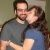# Science Quiz / Solving Compound Inequalities II

Random Science Quiz

## Can you name the answer to these compound inequalities?

#### bydianaburnham08  Plays Quiz not verified by Sporcle

Score
0/10
Timer
20:00
12 < x-10 < 13
a-6 < 3 or a+2 > 15
2w < = 18 and -3w < = 9Use < = for 'Less than or equal to' and Use > = for 'Greater than or equal to'
1/3z < -11 or 1/4z > 2
-4 < = 3t + 2 < 11Use < = for 'Less than or equal to'
4m - 3 > = 9 and -2m+6 > -10Use > = for 'Greater than or equal to'
5p - 2 < = -7 or 7p + 3 > = 17Use < = for 'Less than or equal to' and Use > = for 'Greater than or equal to'
-6 > 2g + 12 > -8
1/3k + 9 < 10 or 1/4k - 2 > 7
8 > 4-1/2j > = 4Use < = for 'Less than or equal to'

## From the Vault See Another

Desert Countries
Dessert Countries would be much sweeter.

### Extras

Created Jan 13, 2013
Tags: